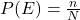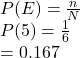## minh has a number cube it has a different number on each side on each side :4,5,6,7,8, or 9. minh will roll the number cube once what is the

Question

minh has a number cube it has a different number on each side on each side :4,5,6,7,8, or 9. minh will roll the number cube once what is the probabillity he will roll a 5?

in progress 0
2 months 2021-07-30T18:26:09+00:00 1 Answers 1 views 0

The probability he will roll a 5 is 0.167.

Step-by-step explanation:

Minh has a 6-number cube it has a different number on each side.

The number on each side are: 4, 5, 6, 7, 8 or 9.

As there are different number on each face of the cube, on every roll the possible outcomes are:

S = {4, 5, 6, 7, 8 or 9}

n (S) = 6

The probability of an event E is the ratio of the favorable number f outcomes to the total number of outcomes.Compute the probability of rolling a 5 as follows:Thus, the probability he will roll a 5 is 0.167.